# Addition strategies

### Addition strategies

In this lesson, we will learn:

• The addition strategies of: (1) using friendly numbers with adjustment, (2) creating friendly numbers using addends, (3) adding on from left to right, and (4) adding by counting on
• Understanding adding by 9

Notes:

• Method 1: change into a "friendly" number and then adjust (by subtracting)
• Round one of the addends to the nearest multiple of 10
• Then adjust by taking away how much you rounded up by to get the answer• Method 2: make a "friendly" number using the addends
• Use the numbers that were given for the addition equation
• Move values from one number to the other number to make a multiple of 10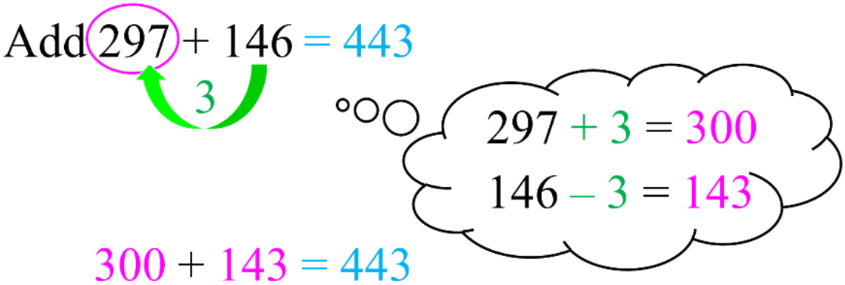• Method 3: adding from left to right
• Add by going through one place value at a time
• i.e. hundreds of 1st # + hundreds 2nd # + tens of 1st # + tens of 2nd # + . . .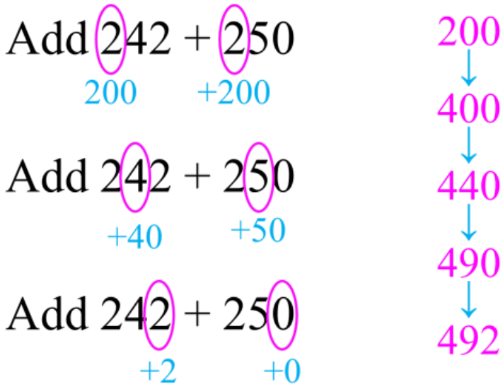• Method 4: adding by counting on
• Start with the first number, then add the second number one place value at a time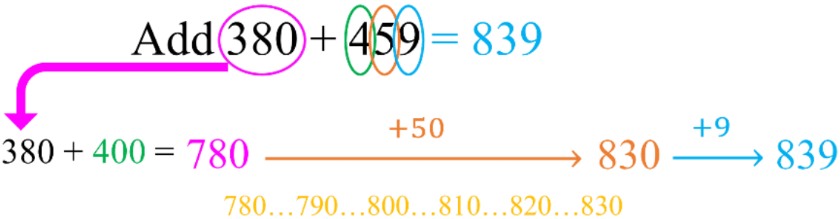#### Lessons

• Introduction
Introduction to Addition Strategies:
a)
Mental math and sums of 10

b)
Addition strategy #1: friendly numbers with adjustment

c)
Addition strategy #2: creating friendly numbers using addends

d)
Addition strategy #3: adding on from left to right

e)
Addition strategy #4: adding by counting on

• 1.
Adding by grouping sums of 10
Add by pairing up sums of 10. Circle the pair and fill in the blanks.
a)
8 + 5 + 2 = ____
10 + ____ = ____

b)
1 + 7 + 3 = ____
10 + ____ = ____

c)
6 + 9 + 4 = ____
10 + ____ = ____

• 2.
Adding by 9 strategy
Add 9 by: adding 10 first, then taking 1 away. Fill in the blanks.
a)
7 + 9 = ____
7 + 10 = ____ - 1 = ____

b)
14 + 9 = ____
14 + 10 = ____ - 1 = ____

c)
38 + 9 = ____
38 + 10 = ____ - 1 = ____

• 3.
Adding by 8 strategy
Add 8 by: adding 10 first, then taking 2 away. Fill in the blanks.
a)
7 + 8 = ____
7 + 10 = ____ - 2 = ____

b)
16 + 8 = ____
16 + 10 = ____ - 2 = ____

c)
54 + 8 = ____
54 + 10 = ____ - 2 = ____

• 4.
Adding using friendly numbers with adjustment
Add by changing one number into a "friendly" number and adjust. Fill in the blanks.
a)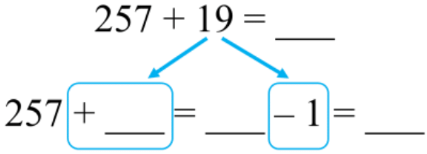b)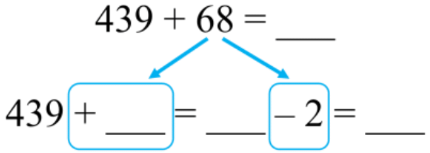c)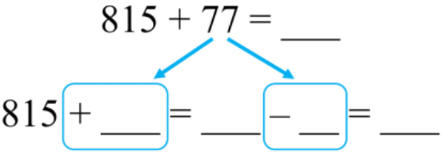• 5.
Adding by making friendly numbers with given addends
Add: make "friendly" numbers using given addends. Fill in the blank.
a)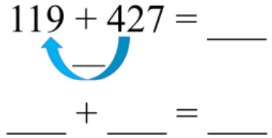b)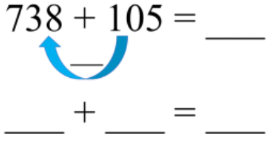c)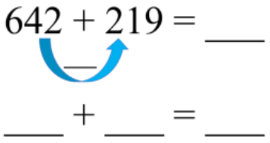• 6.
Adding on strategy
Use the method of adding on from left to right.
a)
553 + 385 = ____

b)
730 + 291 = _____

c)
664 + 148 = ____

• 7.
Counting on from the first number
Add by counting on from the first number
a)
104 + 693 = ____

b)
827 + 335 = _____

c)
568 + 429 = ____